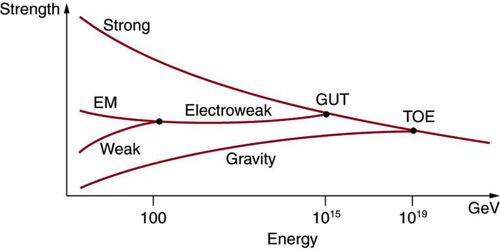# Unification of Forces

Unification of Forces

In nature, we are familiar with different types of forces which have different names as well. But all these forces are not fundamental. At the fundamental levels, all these forces fall into four groups. These forces are called fundamental forces.

In order to establish relations among the four fundamental forces scientists have been working for many years. Previously, electric force and magnetic force were considered as independent fundamental forces. But different experimental results in the nineteenth century indicated that electric and magnetic forces were not independent. Finally, J. C. Maxwell discovered electromagnetic force by which he established a relation between electric and magnetic forces.

Most physicists consider that the three basic forces, gravitation, electroweak, and nuclear force, come from a generic force. Since we do not know the origin of these three basic forces, this assertion is pure speculation.

Salam, Wienberg, and Glashow have recently established a relation between weak nuclear force and the electromagnetic force. Scientists are working hard to establish a grand held theory which will unify all the fundamental forces into one force.

Weinberg and Salam (Nobel Prize 1979) proved that the weak nuclear force is nothing but the EM force. These two have been merged into a common force called “Electroweak Force”.

The Spacetime Model goes further demonstrating that EM waves, W or Z bosons, protons, neutrons, electrons, gluons, and all basic elements are always made of spacetime.